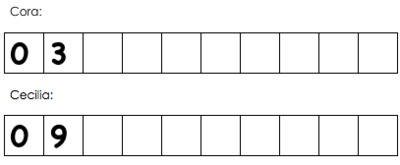# Patterns and the Coordinate Plane

## Objective

Generate two numerical patterns using two given rules, plotting the points, and identify relationships between corresponding terms.

## Common Core Standards

### Core Standards

?

• 5.OA.B.3 — Generate two numerical patterns using two given rules. Identify apparent relationships between corresponding terms. Form ordered pairs consisting of corresponding terms from the two patterns, and graph the ordered pairs on a coordinate plane. For example, given the rule "Add 3" and the starting number 0, and given the rule "Add 6" and the starting number 0, generate terms in the resulting sequences, and observe that the terms in one sequence are twice the corresponding terms in the other sequence. Explain informally why this is so.

?

• 4.OA.C.5

## Criteria for Success

?

1. Generate two numerical patterns given their rules.
2. Understand that a term is a number in a pattern and corresponding terms are numbers in two patterns that show up in the same place in each pattern (e.g., the second term of each pattern are corresponding terms).
3. Form coordinate pairs consisting of corresponding terms from the two patterns.
4. Graph the ordered pairs in a coordinate plane.
5. Look for and express repeated reasoning to identify relationships between corresponding terms (MP.8).
6. Explain why those relationships between corresponding terms exist (MP.3).

## Tips for Teachers

?

#### Remote Learning Guidance

If you need to adapt or shorten this lesson for remote learning, we suggest prioritizing Anchor Task 1 (benefits from discussion) and Anchor Task 2 (benefits from worked example). Find more guidance on adapting our math curriculum for remote learning here.

#### Fishtank Plus

• Problem Set
• Student Handout Editor
• Vocabulary Package

?

### Problem 1

Melissa and Joe are reading their books during independent reading. Because Joe’s book has more pictures in it, he can read more pages in the same amount of time. When Melissa is done with 2 pages of reading, Joe is done with 4 pages.

1. Complete the table below to see how many pages Joe and Melissa will each read.
 Number of Pages Melissa Reads Number of Pages Joe Reads 2 4 6 8 10 12
1. Create a coordinate graph using the information in the table above.
2. What do you notice about the number of pages Melissa and Joe will read? What do you wonder?

### Problem 2

Xavier is making two different patterns. Pattern P has a starting number of 0 and he adds 12 each time. Pattern Q also has a starting number of 0, but he adds 3 each time.

1. Generate the first 10 terms, or numbers in the pattern, of Pattern P and Pattern Q
2. Create a coordinate graph using Patterns P and Q
3. How many times greater is the third term in Pattern P than the third term in Pattern Q? What about the seventh term?
4. Without extending the pattern, how many times greater will the 20th term in Pattern P be than the 20th term in Pattern Q? How do you know?
5. Why does this relationship between Pattern P and Q exist?

## Problem Set & Homework

#### Discussion of Problem Set

• What is the relationship between the x- and y-coordinates on the coordinate plane in #2? How do you see that pattern in the coordinate grid? How would you see it in a table?
• What relationships did you notice in the coordinate pairs in #3(e)? Why did these relationships exist?
• How did the relationship between corresponding terms in #3 differ from the relationship between corresponding terms in #4?
• What was the relationship between corresponding terms in #5? How was this different than the other pairs of patterns we’ve looked at today?

?

### Problem 1

Lars wrote rules for two patterns.

 Pattern for x-values Pattern for y-values Starting number =1 Starting number =1 Rule: Add 2 Rule: Multiply by 2

Lars then wrote ordered pairs (xy) using the patterns above. Which ordered pair could Lars have written?

1. (2, 3)
2. (3, 2)
3. (3, 6)
4. (5, 5)

#### References

Page 37

From EngageNY.org of the New York State Education Department. New York State Testing Program Grade 6 Common Core Mathematics Test Released Questions with Annotations August 2014. Internet. Available from https://www.engageny.org/resource/new-york-state-common-core-sample-questions; accessed May 23, 2018, 12:20 p.m..

### Problem 2

Cora and Cecilia each use chalk to make their own number patterns on the sidewalk. They make each of their patterns 10 boxes long and line their patterns up so they are next to each other.

• Cora puts 0 in her first box and decides that she will add 3 every time to get the next number.
• Cecilia puts 0 in her first box and decides that she will add 9 every time to get the next number.1. Complete each girl's sidewalk pattern.
2. How many times greater is Cecilia’s number in the fifth box than Cora’s number in the fifth box?  What about the numbers in the eighth box? The tenth box?
3. What pattern do you notice in your answers for part (b)?  Why do you think that pattern exists?

#### References

Illustrative Mathematics Sidewalk Patterns

Sidewalk Patterns, accessed on May 23, 2018, 12:21 p.m., is licensed by Illustrative Mathematics under either the CC BY 4.0 or CC BY-NC-SA 4.0. For further information, contact Illustrative Mathematics.

Modified by The Match Foundation, Inc.

### Mastery Response

?